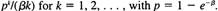### Create an Account

Home / Questions / Let X t be a Yule process that is observed at a random time U where U is uniformly distributed over

# Let X t be a Yule process that is observed at a random time U where U is uniformly distributed over

Let X(t) be a Yule process that is observed at a random time U, where U is uniformly distributed over [0, 1). Show that Pr{X(U) = k} =Aug 04 2020 View more View LessSubscribe To Get Solution